What are the different types of angles?

• Last Updated : 17 Aug, 2021

Geometry had played an essential role in the development of the modern world. During the time geometry is used for designing, constructional works, architecture in choice of material for construction, and many more. It is also a vital part of the technical background for computing various designs, manufacturing, creating blueprints, programming, etc.

Geometry is a branch of mathematics that deals with the study of shapes and their properties.

The approach for geometry can be observed from ancient times in their constructions from the use of various shapes in a very specific way. The term is originally derived from Greek words ‘ge’ and ‘materia’ which means earth and measurement respectively.

The given article is a study about angles, and their various types based on their measurements.

Angles

Angles can be simply defined as is the space between two intersecting lines meeting at a certain point. They consist of two arms known as sides of the angle and a meeting point where angle forms called a vertex. Angles are measured in degrees which measures from 0° to 360°.

An Angle is a shape or space formed at the meeting point of two intersecting rays.

The word angle is derived from the Latin word ‘angulus’ which means ‘a corner. The first angle was supposed by Carpus of Antioch.

Angles are divided into different types on the basis of measurement and rotation.

Types of Angles

Angles are divided into multiple types i.e. on the basis of Measurement and on the basis of rotation, that is:

Angles on the basis of measurement:

Acute angle: The angle that measures less than 90° is the acute angle. The degree always measures between 0 and 90. Acute angles measure positive while the rotation is anticlockwise and negative when the rotation of angle is clockwise.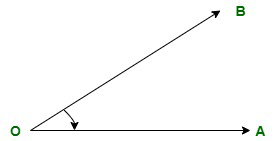In the figure, O is the vertex of the angle, and OA and OB are two intersecting rays that meet at point O forming an angle less than 90°. Hence, ∠AOB is an acute angle.

Right angle: The angle that exactly measures 90° is a right angle. It is also considered as a half straight angle as half of 180° makes a right angle. The value of the angle may be positive or negative on the basis of the rotation of the angle.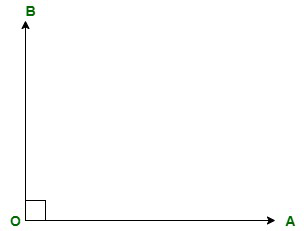In the figure, O is the vertex of the angle, and OA and OB are two intersecting rays that meet at point O forming an angle of exactly 90°. Hence, ∠AOB is a right angle.

Obtuse angle: The angle that measures more than 90° and less than 180° is an obtuse angle. The degree always lies between 90° and 180°. The value of the obtuse angle will be positive if the rotation is anticlockwise and negative if the rotation is clockwise.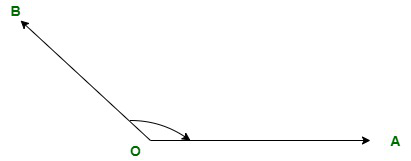In the figure, O is the vertex of the angle, and OA and OB are two intersecting rays that meet at point O forming an angle of more than 90°. Hence, ∠AOB is an obtuse angle.

Straight angle: The angle that measures exactly 180° is a straight angle. Straight angles form straight lines. The measure of straight angle can be positive or negative. If we move  anticlockwise the straight angle will measure 180° and if we move clockwise the angle will measure -180°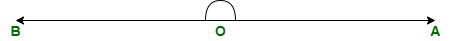In the figure, of a straight angle, we can observe that O is the meeting point of two arms that is called vertex and OA and OB are two sides of the angle.

Reflex angle: The angle that measures more than 180° and less than 360° is the reflex angle. The degree always lies between 180° and 360°.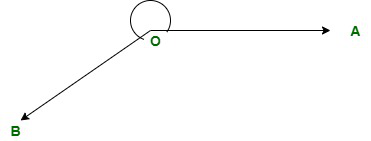In the figure, O is the vertex of the angle, and OA and OB are two intersecting rays that meet at point O forming an angle of more than 180°. Hence, ∠AOB is a reflex angle.

Angles on the basis of rotation:

• Positive angles: The angle that moves anticlockwise from its base and is drawn from the point (x, y) which is its origin is a positive angle.
• Negative angles: The angle that moves clockwise from its base and is drawn from the point (-x, -y)  which is its origin is a negative angle.

Complementary Angles

If the summation of two angles measures 90° then, the angle is said to be a complementary angle. The two angles combining together does not require to be adjacent or similar. It can be any two types of angles measuring 90° after addition.

In the given figure (i), ∠AOC and ∠COB are two adjacent acute angles with the measure of 45°. And, their sum is equal to 90° which means it is a complementary angle.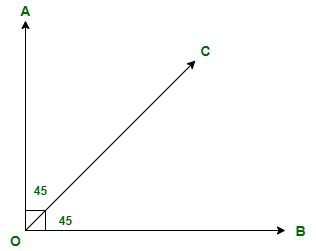In figure (ii), ∠AOC and ∠COB are two angles measuring 70° and 20° respectively. The two angles are not adjacent to each other but their sum equals 90° hence, it is also a complementary angle.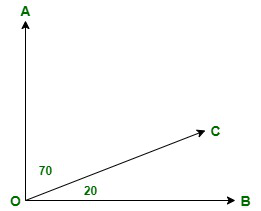Supplementary Angles

If the summation of two angles measures 180°, the angle is said to be a supplementary angle. Supplementary angles are further categorized into four types which are briefly discussed below:

Vertical angle: When two angles combining together have the same vertex and are equal to each other then it is a vertical angle.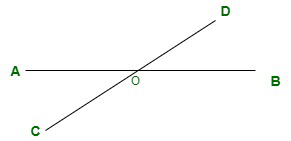In the above figure, ∠AOD and ∠COB are vertical angles. Similarly, ∠AOC and ∠BOD are also vertical angles present opposite each other.

Alternate Interior angle: The angles which are present on opposite sides of the transversal is the alternative interior angle. They are present at the inner side of the Z formed in the figure. Both the angles are equal to each other.

The figure we have been given below is a combination of different angles. In the figure, ∠AOT and ∠OTR are alternate interior angles placed interiorly alternate to each other. Similarly, ∠BOT and ∠OTQ are also interior angles.

Alternative Exterior angle: The angles which are present on opposite sides of the transversal but externally are the alternative exterior angle. They are spotted at the exterior part and both the angles measure the same.

In the figure, ∠SOB and ∠QTP, ∠SOA, and ∠RTP are the pair of alternate exterior angles.

Corresponding angle: The angles that are present at similar positions are corresponding angles. Both the angles measure the same.

In the figure, ∠SOB and ∠OTR are corresponding angles place at similar positions one at the exterior and the other in the interior part.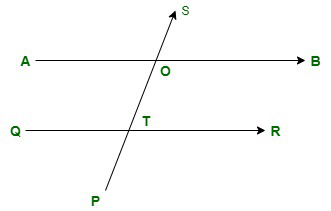Sample Questions

Question 1: Which of the angles form a straight line?

The straight angle with a measurement of 180 degrees forms a straight line with two endpoints.

Question 2: Which of the angle is also known as half turn?

The straight angle is known as half turn as the sum of two straight angles forms 360° which is a full turn angle.

Question 3: List the measurements of all the angles.

Acute angle: less than 90°

Right Angle: exactly 90°

Obtuse angle: more than 90° and less than 180°

Straight angle: exactly 180°

Reflex angle: more than 180° and less than 360°

Question 4: Can angles be negative?